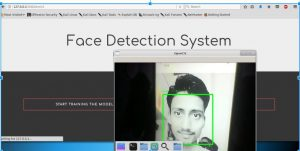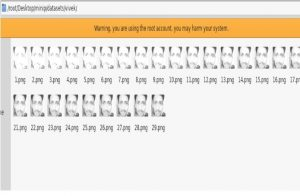# Face Detection using Python and OpenCV with webcam

OpenCV is a Library which is used to carry out image processing using programming languages like python. This project utilizes OpenCV Library to make a Real-Time Face Detection using your webcam as a primary camera.

Following are the requirements for it:-

1. Python 2.7
2. OpenCV
3. Numpy
4. Haar Cascade Frontal face classifiers

Approach/Algorithms used:

1. This project uses LBPH (Local Binary Patterns Histograms) Algorithm to detect faces. It labels the pixels of an image by thresholding the neighborhood of each pixel and considers the result as a binary number.
2. LBPH uses 4 parameters :
(i) Radius: the radius is used to build the circular local binary pattern and represents the radius around the
central pixel.
(ii) Neighbors : the number of sample points to build the circular local binary pattern.
(iii) Grid X : the number of cells in the horizontal direction.
(iv) Grid Y : the number of cells in the vertical direction.
3. The model built is trained with the faces with tag given to them, and later on, the machine is given a test data and machine decides the correct label for it.

How to use :

1. Create a directory in your pc and name it (say project)
2. Create two python files named create_data.py and face_recognize.py, copy the first source code and second source code in it respectively.
3. Copy haarcascade_frontalface_default.xml to the project directory, you can get it in opencv or from
here.
4. You are ready to now run the following codes.

 `# Creating database ` `# It captures images and stores them in datasets  ` `# folder under the folder name of sub_data ` `import` `cv2, sys, numpy, os ` `haar_file ``=` `'haarcascade_frontalface_default.xml'` ` `  `# All the faces data will be ` `#  present this folder ` `datasets ``=` `'datasets'`   ` `  ` `  `# These are sub data sets of folder,  ` `# for my faces I've used my name you can  ` `# change the label here ` `sub_data ``=` `'vivek'`      ` `  `path ``=` `os.path.join(datasets, sub_data) ` `if` `not` `os.path.isdir(path): ` `    ``os.mkdir(path) ` ` `  `# defining the size of images  ` `(width, height) ``=` `(``130``, ``100``)     ` ` `  `#'0' is used for my webcam,  ` `# if you've any other camera ` `#  attached use '1' like this ` `face_cascade ``=` `cv2.CascadeClassifier(haar_file) ` `webcam ``=` `cv2.VideoCapture(``0``)  ` ` `  `# The program loops until it has 30 images of the face. ` `count ``=` `1` `while` `count < ``30``:  ` `    ``(_, im) ``=` `webcam.read() ` `    ``gray ``=` `cv2.cvtColor(im, cv2.COLOR_BGR2GRAY) ` `    ``faces ``=` `face_cascade.detectMultiScale(gray, ``1.3``, ``4``) ` `    ``for` `(x, y, w, h) ``in` `faces: ` `        ``cv2.rectangle(im, (x, y), (x ``+` `w, y ``+` `h), (``255``, ``0``, ``0``), ``2``) ` `        ``face ``=` `gray[y:y ``+` `h, x:x ``+` `w] ` `        ``face_resize ``=` `cv2.resize(face, (width, height)) ` `        ``cv2.imwrite(``'% s/% s.png'` `%` `(path, count), face_resize) ` `    ``count ``+``=` `1` `     `  `    ``cv2.imshow(``'OpenCV'``, im) ` `    ``key ``=` `cv2.waitKey(``10``) ` `    ``if` `key ``=``=` `27``: ` `        ``break`

Following code should be run after the model has been trained for the faces :

 `# It helps in identifying the faces ` `import` `cv2, sys, numpy, os ` `size ``=` `4` `haar_file ``=` `'haarcascade_frontalface_default.xml'` `datasets ``=` `'datasets'` ` `  `# Part 1: Create fisherRecognizer ` `print``(``'Recognizing Face Please Be in sufficient Lights...'``) ` ` `  `# Create a list of images and a list of corresponding names ` `(images, lables, names, ``id``) ``=` `([], [], {}, ``0``) ` `for` `(subdirs, dirs, files) ``in` `os.walk(datasets): ` `    ``for` `subdir ``in` `dirs: ` `        ``names[``id``] ``=` `subdir ` `        ``subjectpath ``=` `os.path.join(datasets, subdir) ` `        ``for` `filename ``in` `os.listdir(subjectpath): ` `            ``path ``=` `subjectpath ``+` `'/'` `+` `filename ` `            ``lable ``=` `id` `            ``images.append(cv2.imread(path, ``0``)) ` `            ``lables.append(``int``(lable)) ` `        ``id` `+``=` `1` `(width, height) ``=` `(``130``, ``100``) ` ` `  `# Create a Numpy array from the two lists above ` `(images, lables) ``=` `[numpy.array(lis) ``for` `lis ``in` `[images, lables]] ` ` `  `# OpenCV trains a model from the images ` `# NOTE FOR OpenCV2: remove '.face' ` `model ``=` `cv2.face.LBPHFaceRecognizer_create() ` `model.train(images, lables) ` ` `  `# Part 2: Use fisherRecognizer on camera stream ` `face_cascade ``=` `cv2.CascadeClassifier(haar_file) ` `webcam ``=` `cv2.VideoCapture(``0``) ` `while` `True``: ` `    ``(_, im) ``=` `webcam.read() ` `    ``gray ``=` `cv2.cvtColor(im, cv2.COLOR_BGR2GRAY) ` `    ``faces ``=` `face_cascade.detectMultiScale(gray, ``1.3``, ``5``) ` `    ``for` `(x, y, w, h) ``in` `faces: ` `        ``cv2.rectangle(im, (x, y), (x ``+` `w, y ``+` `h), (``255``, ``0``, ``0``), ``2``) ` `        ``face ``=` `gray[y:y ``+` `h, x:x ``+` `w] ` `        ``face_resize ``=` `cv2.resize(face, (width, height)) ` `        ``# Try to recognize the face ` `        ``prediction ``=` `model.predict(face_resize) ` `        ``cv2.rectangle(im, (x, y), (x ``+` `w, y ``+` `h), (``0``, ``255``, ``0``), ``3``) ` ` `  `        ``if` `prediction[``1``]<``500``: ` ` `  `           ``cv2.putText(im, ``'% s - %.0f'` `%`  `(names[prediction[``0``]], prediction[``1``]), (x``-``10``, y``-``10``),  ` `cv2.FONT_HERSHEY_PLAIN, ``1``, (``0``, ``255``, ``0``)) ` `        ``else``: ` `          ``cv2.putText(im, ``'not recognized'``,  ` `(x``-``10``, y``-``10``), cv2.FONT_HERSHEY_PLAIN, ``1``, (``0``, ``255``, ``0``)) ` ` `  `    ``cv2.imshow(``'OpenCV'``, im) ` `     `  `    ``key ``=` `cv2.waitKey(``10``) ` `    ``if` `key ``=``=` `27``: ` `        ``break`

Note : Above programs will not run on online IDE.

Screenshots of the Program

It may look something different because I had integrated the above program on flask framework

Running of second program yields results similar to the below image :face detection

Datasets Storage :data_sets

Attention geek! Strengthen your foundations with the Python Programming Foundation Course and learn the basics.

To begin with, your interview preparations Enhance your Data Structures concepts with the Python DS Course.

My Personal Notes arrow_drop_updie with memories not dreams

If you like GeeksforGeeks and would like to contribute, you can also write an article using contribute.geeksforgeeks.org or mail your article to contribute@geeksforgeeks.org. See your article appearing on the GeeksforGeeks main page and help other Geeks.

Please Improve this article if you find anything incorrect by clicking on the "Improve Article" button below.

Article Tags :

7

Please write to us at contribute@geeksforgeeks.org to report any issue with the above content.